## Harry Potter Word2Vec

Ever wonder what the computer thinks when it "reads" a book? Well, in this post we will be using Natural Language Processing (NLP) to analyze the text in J.K. Rowling's Harry Potter and the Philosopher's Stone. In the field of machine learning, data is often represented in higher dimensions. For example, when we think of a person, we often have mental representation of the person's features. Is he a male or a female? How old is he/she? How tall? And you can think of a million other things that could be features of a person. To represent these features mathematically, data scientists often use vectors or matrices. A vector is a collection of numbers, and the number of numbers in this vector is easily thought of as the dimension of the vector. Why represent data as vectors? When we represent data as a mathematical object, it allows us to easily apply mathematical properties or algorithms to extract information out of the data.

#### NLP - A Machine Learning Approach

So you may ask, "if I have data as unstructured as a corpus or a book, how do I even go about representing that object mathematically as you just mentioned?" Well, you are lucky because there's an algorithm that does just that for you! The algorithm's called Word2Vec. As the name suggests, it converts words in a corpus into vectors that we "hope" would contain some information about the words. Because we are doing machine learning, we can save some work by letting the machine do the work. In general, Machine Learning works by:

1. Specifying the objective to the computer program, where the input to this program is our data
2. Solve for a mathematical formula that gives the program a direction to update its parameters, based on that objective
3. Run the program until the parameters no longer updates (it's reached convergence)

#### Word2Vec Algorithm

In our case, there's an algorithm to do that, called the Skip-Gram algorithm. The Skip-Gram model is based on the belief that the denotation, the connotation, or the literary elements of each word can be understood from its context - the words around our word of interest. With that, we have the objective function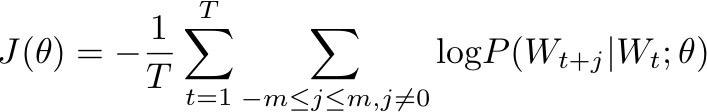which is the maximum likelihood (or the most probable distribution with the set of given parameters) of the event where the words and context appear the way they do in this corpus. It is mathematically equivalent to minimize the negative log-likelihood:What are the probabilities P(.) above? Machine learning practitioners often use the softmax function which takes in an input and "squashes" it into a probability space where the output is between 0 and 1. The softmax function:Finally, let us define two more mathematical objects to complete our model.In this window, fox would be our center word, and ["the", "quick", "brown", "jumps", "over"] would be our outside words. These words are represented by the dot product of their u's and v's depending on their location in the sliding window.

Where these vectors are vector representations of a word in our corpus. We can take the dot product of these two vectors (dot products give a measure of similarity in the vector space), so we can treat the resulting product as a representation of the word of interest.These u's and v's are vectors of high dimension where data scientists tune the dimension to best fit the dataset. These probabilities are then plugged into our formula for J above to get the full objective function. Now we have gotten our objective, which completes the first step in building a machine learning system!

The second part then involves solving for the gradient of the objective function with respect to our word vectors. From there, we can apply gradient descent to update the word vectors:where theta here represents the vector representations of all our words, alpha the learning rate, and the last term being the gradient of our object function with respect to the word vectors (step 2 of machine learning!). Finally we run the computer program to keep doing this update until it converges (step 3). This idea is so remarkable and powerful because it allows the computer to learn all the "hidden factors" that drives the words to have their denotation, connotation, literary elements, etc. that contribute to its place and context of this corpus, without us knowing what each hidden factor represents!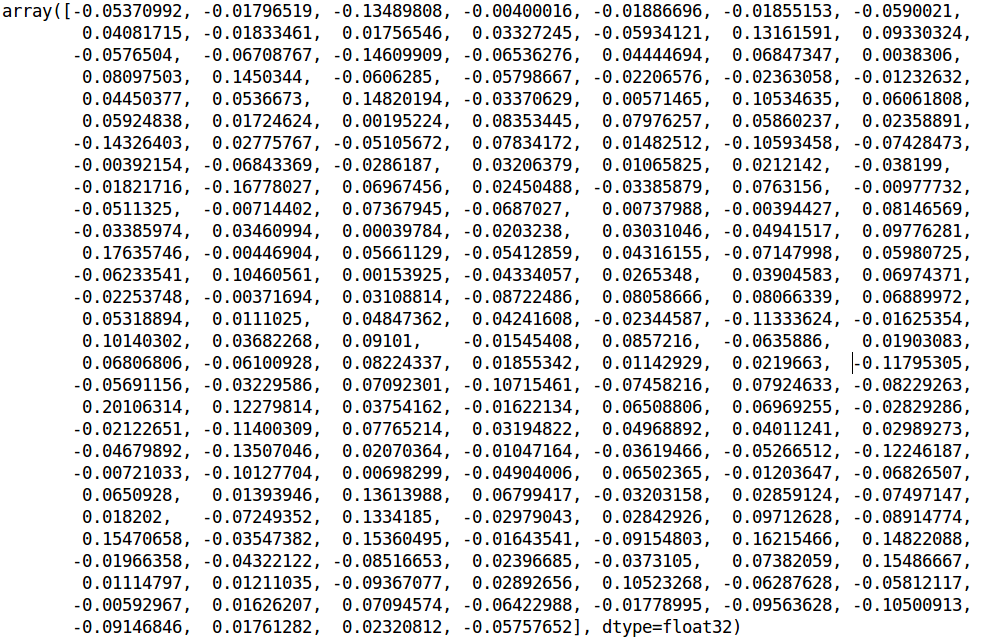An example of what a word might look like in higher dimension…!

#### Learned Vectors

Dealing with high-dimensional data may be irritating. Although the vectors contain information that may be useful for us, it's hard for us to get a little clue as to what these information these vectors may be encoding. It is often very useful to do dimension reduction of high-dimensional objects. This allows us to visualize the location of the objects with respect to each other, had the information been summarized in a lower dimension where humans can comprehend.

Two extremely popular algorithms are Principal Component Analysis (PCA) and t-distributed Stochastic Neighbor Embedding (t-SNE). Whereas PCA reduces the dimensionality by finding the linear basis, t-SNE is a non-linear algorithm, which allows the low dimensional basis of the data to be represented more flexibly and without loss of generality.

#### t-SNE

We have vectors x1,…, xN in a high dimension. We want to represent that data in a lower, say d = 2. We first start by "embedding" the notion of similarity between any two points by defining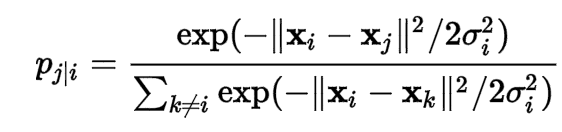to be the conditional probability of point j given point i, using the Gaussian kernel as the similarity measure between two points. This essentially gives us a similarity measure between all pairs of points in the high dimensional space, represented as a probability distribution.

Now we want to represent that similarity in a lower dimension. Let us define the low-dimensional representation of our data with y1,…, yN (say d = 2). We wish to preserve the similarity between every pair of points in this two dimensional space. Define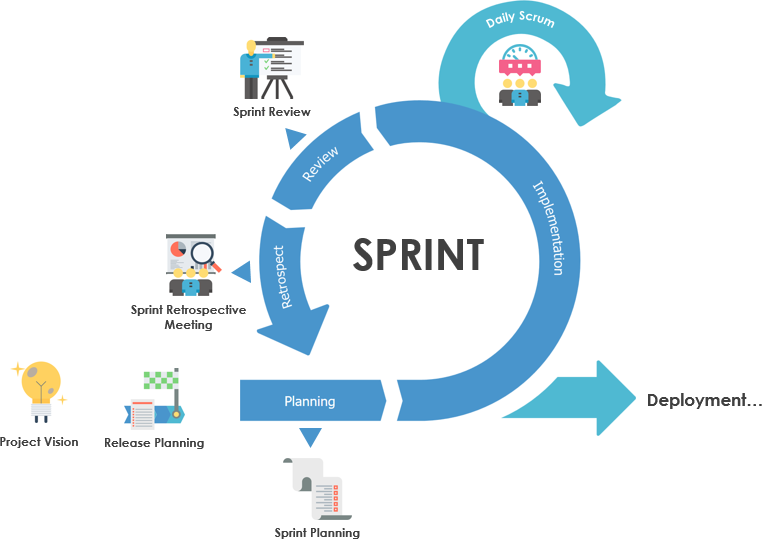as our similarity measure of each point in the low-dimensional vector space. The term in the parenthesis is the kernel of the t-distribution with degree of freedom

1. Again, the q_ij's essentially give us a probability distribution which summarizes the location of each observation in the low-dimensional vector space.

Same as before, with machine learning we always have to define an objective function we wish to optimize over. In this case, we have two probability distributions that summarize the similarity measure in their respective vector space. One measure of the difference between two distributions is the Kullback-Leibler (KL) divergence - we use gradient descent to minimize this objective: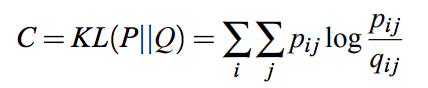For convenience, the gradient of this objective function is given below: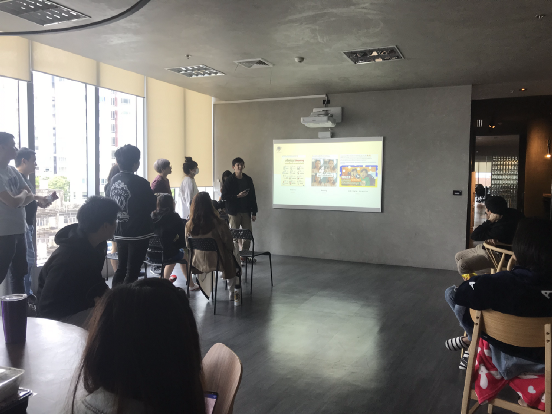The result of t-SNE is then a set of vectors in a low-dimension, where each points have pair-wise similarity measure close to their relative pairs in the high-dimension.

#### The Visualization

Let us plot the reduced vectors in a 2-D plane. In a lower-dimension, we start to see vectors that are similar to cluster together. For example, the similarity of the word "breezy" and "Christmas" is 0.999984 in the high dimension. In the 2-D space, the similarity between these two words is 0.961005. Words like "awkward" and "Christmas" (as you may already feel their sentimental difference) has a similarity score of -0.999893 in the original space, and -0.679286 in the 2-D space.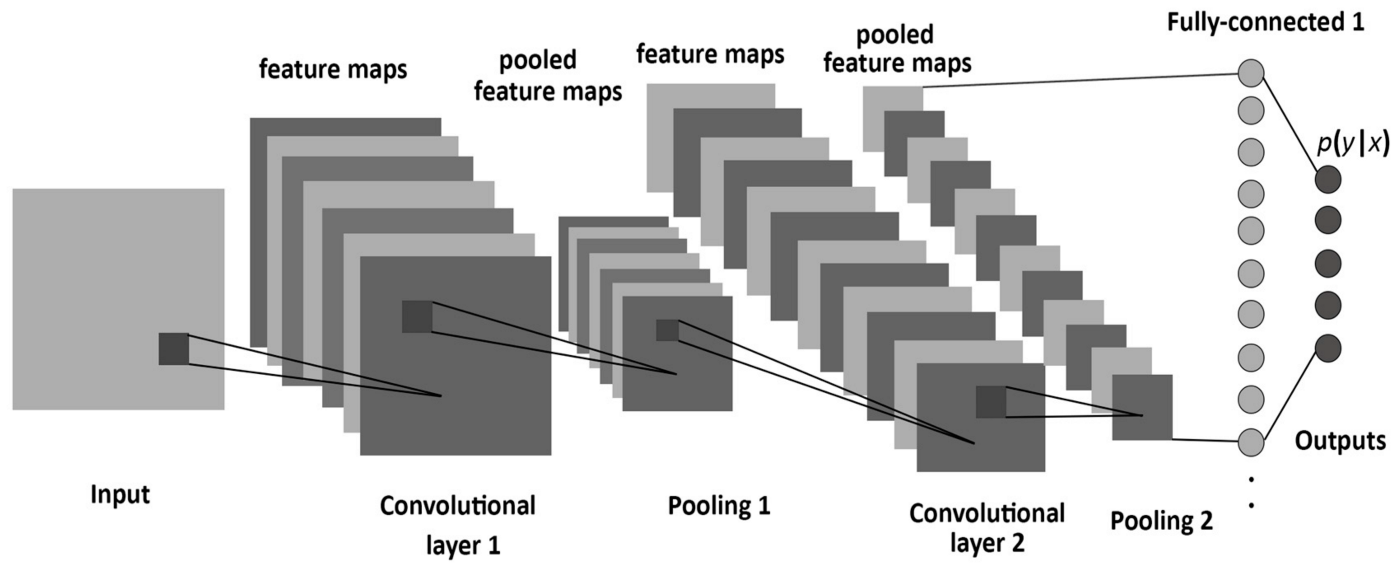#### Conclusion

Word2Vec is a powerful algorithm that allows us to embed words in a corpus into a higher dimensional object - mathematically called "latent variables" - that may encode information about our data. This exercise lays an important foundation for many other NLP tasks because it allows us to cheaply convert words into vectors that may have important features impossible to represent in low-dimensions (a small set of numbers). Not only do these vectors encode a lot of information about the words and their relationship to other words ("context") in the corpus, it is possible to use them as inputs to other powerful algorithms to learn more relationships about their features. For example, we may use an SVM or a Neural Network to classify a word or group of words into different classes (e.g. verbs, nouns, adjectives; violent, peaceful). In other words, this unsupervised learning method allows us to transform an unstructured data, without the need of labels, into mathematical objects that allow us to do many other things. This opens up a whole lot of opportunities for us to do other Deep Learning tasks with natural languages!

## Have a question?

Drop us a line and we will get back to you